# RD Sharma Solutions for Class 10 Maths Chapter 3 Pair of Linear Equations in Two Variables Exercise 3.5

A given system of equations may or may not have a solution. Sometimes, it can also have infinitely many solutions. All these conditions for solvability are studied in this exercise. The RD Sharma Solutions Class 10, prepared by experts at BYJU’S, can help students get a strong conceptual knowledge of the subject. Also, the RD Sharma Solutions for Class 10 Maths Chapter 3 Pair of Linear Equations in Two Variables Exercise 3.5 PDF given below is available for students for further clarification.

## RD Sharma Solutions for Class 10 Maths Chapter 3 Pair of Linear Equations in Two Variables Exercise 3.5 Download the PDF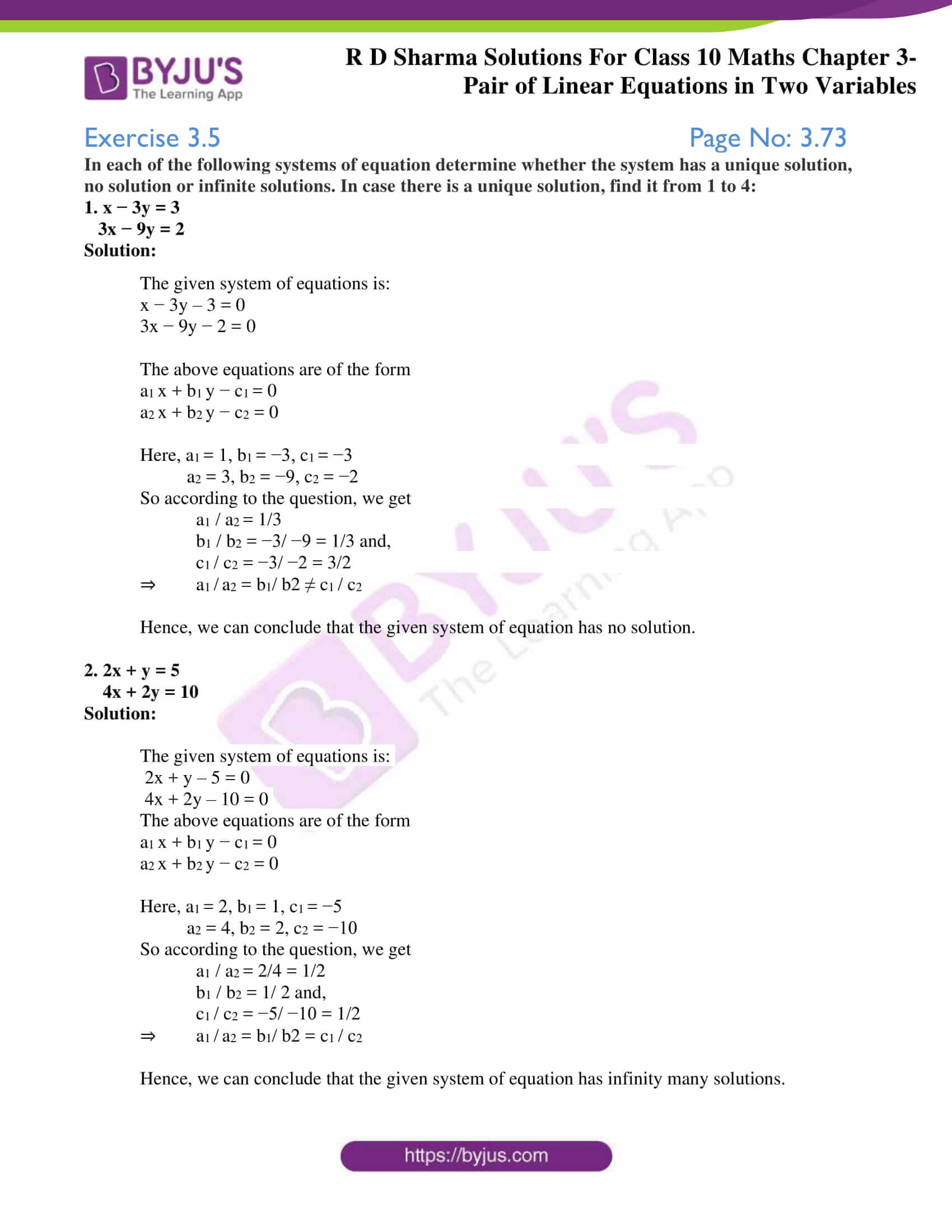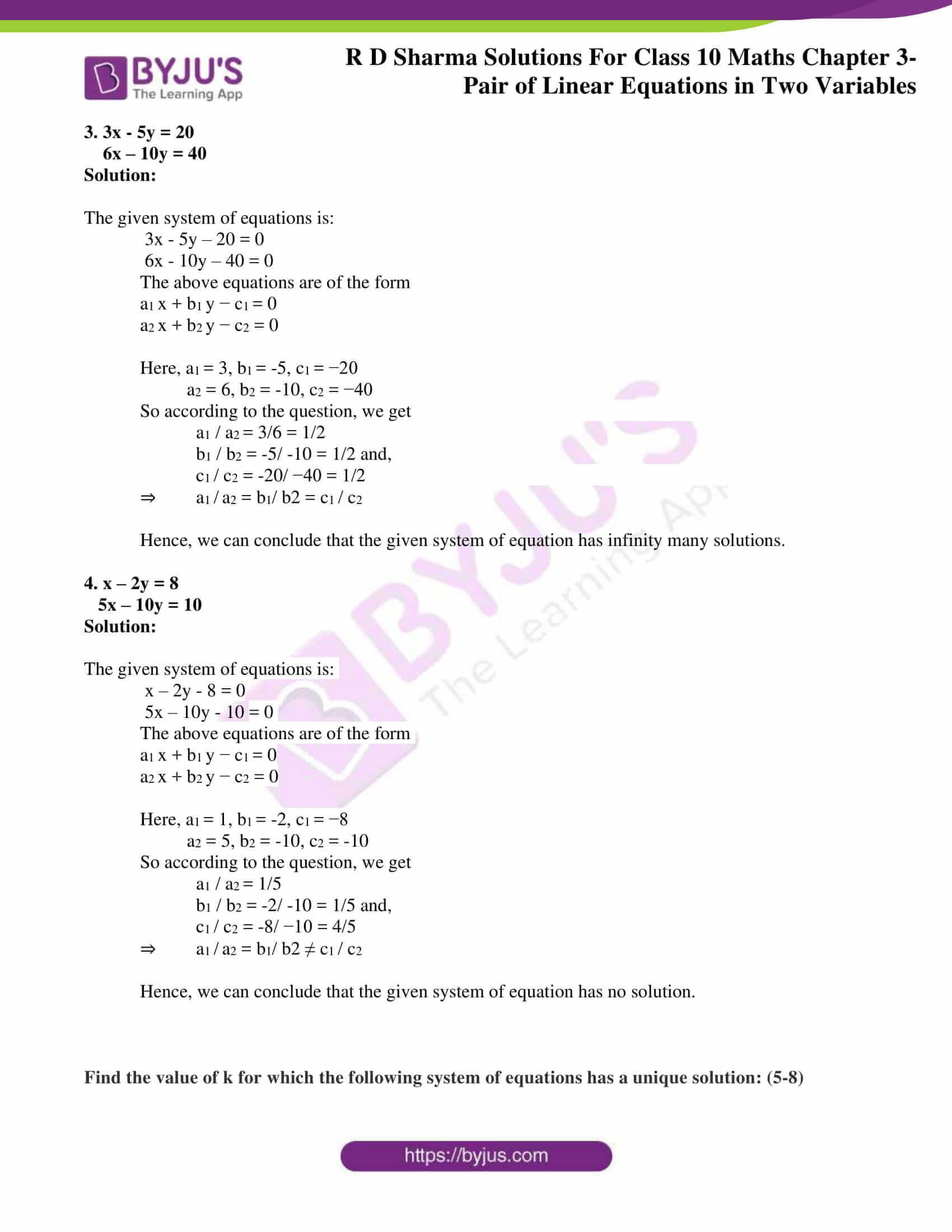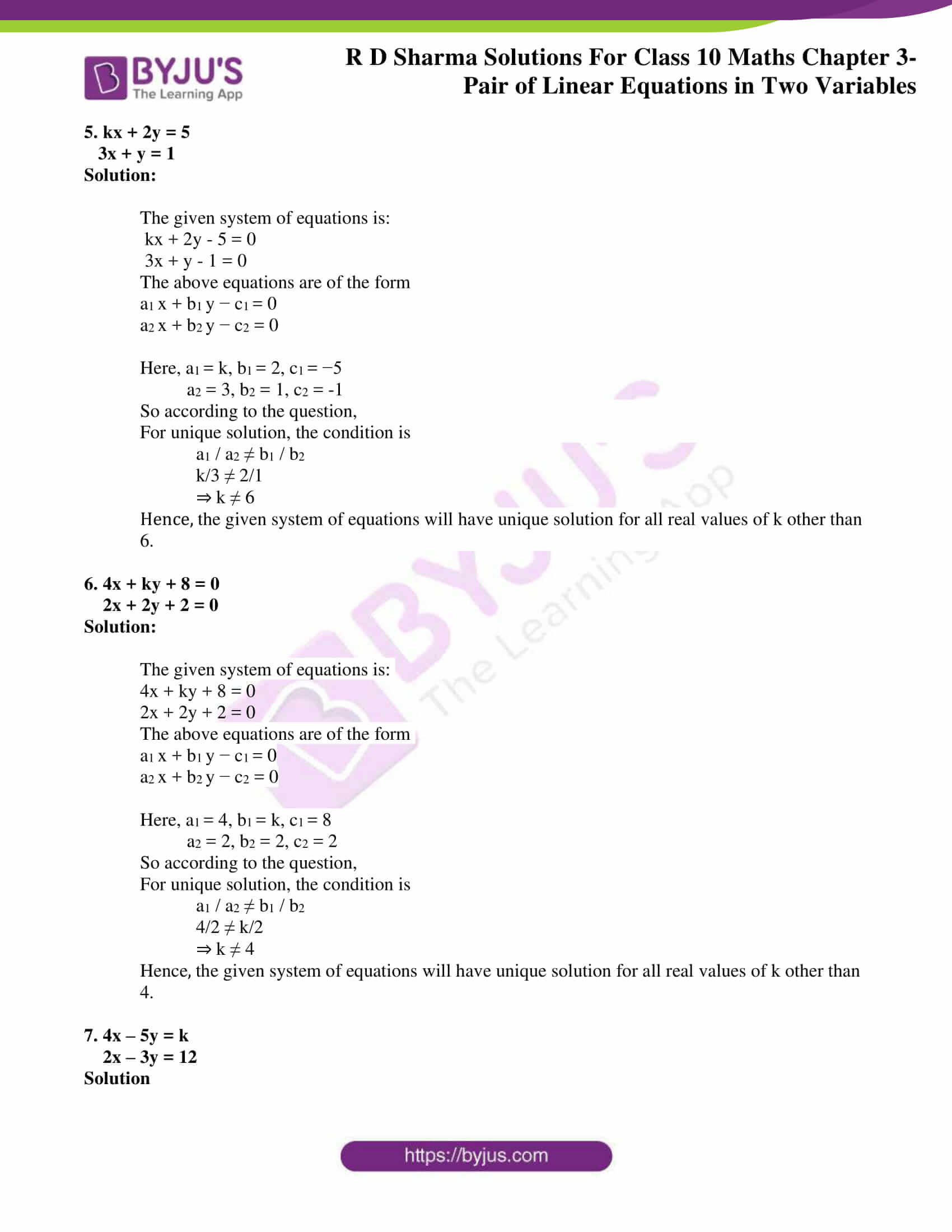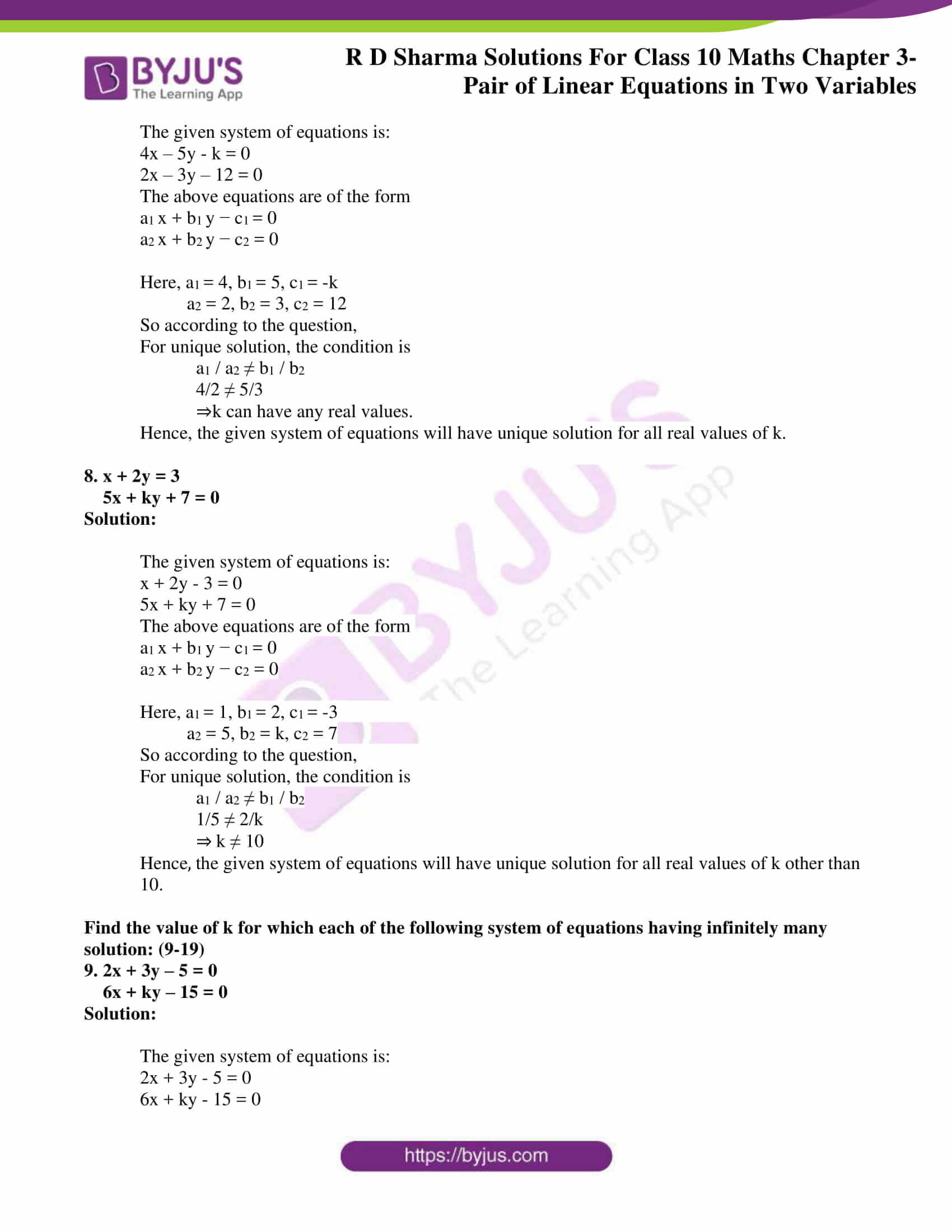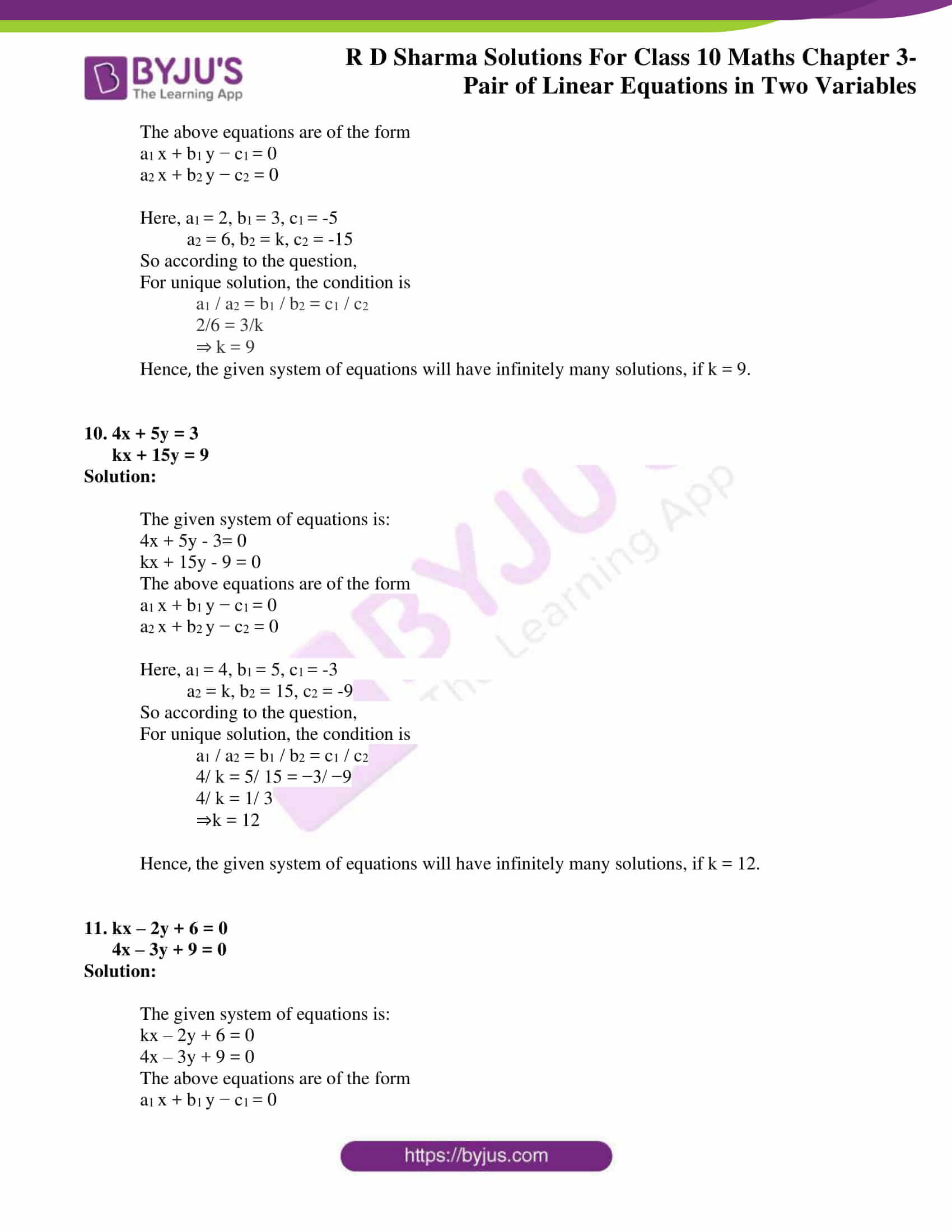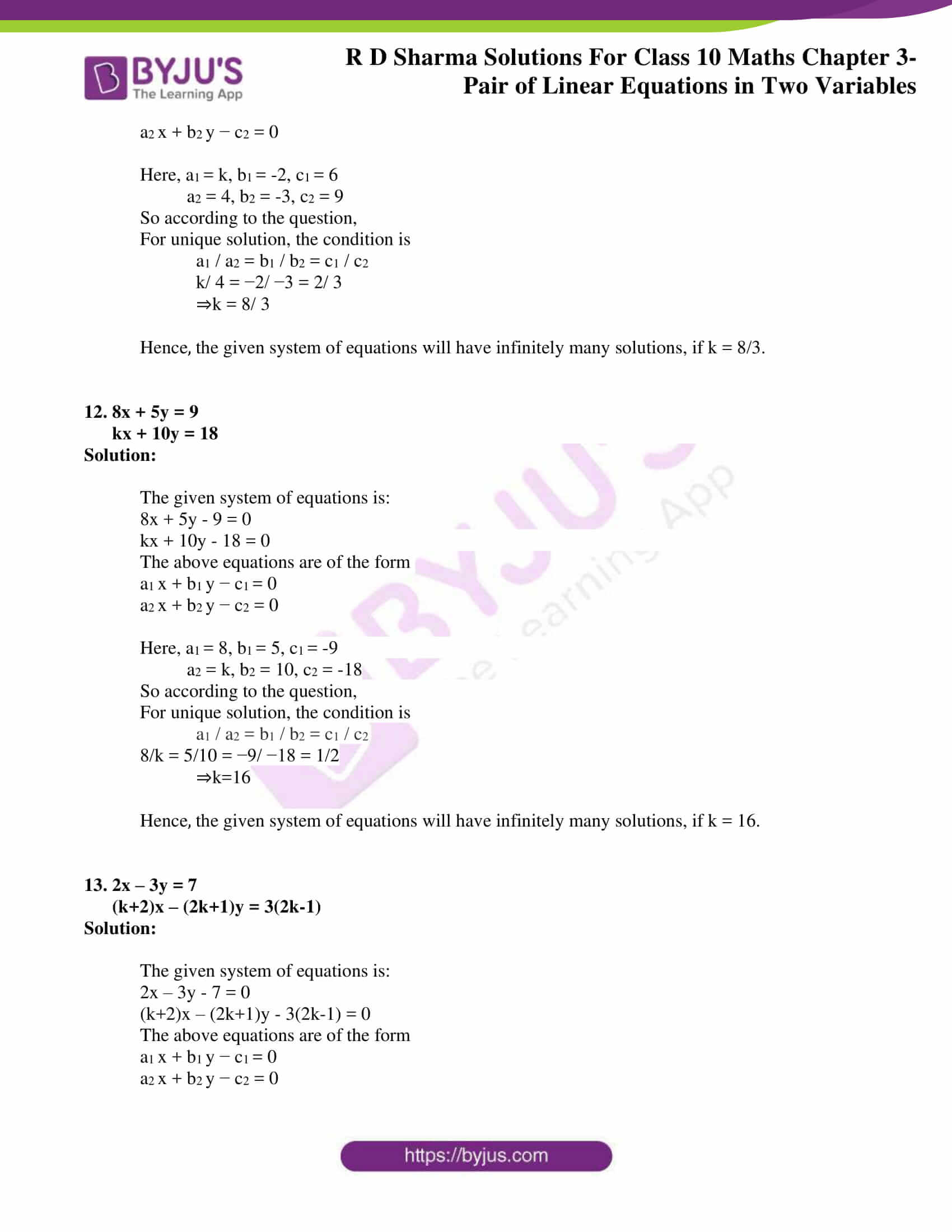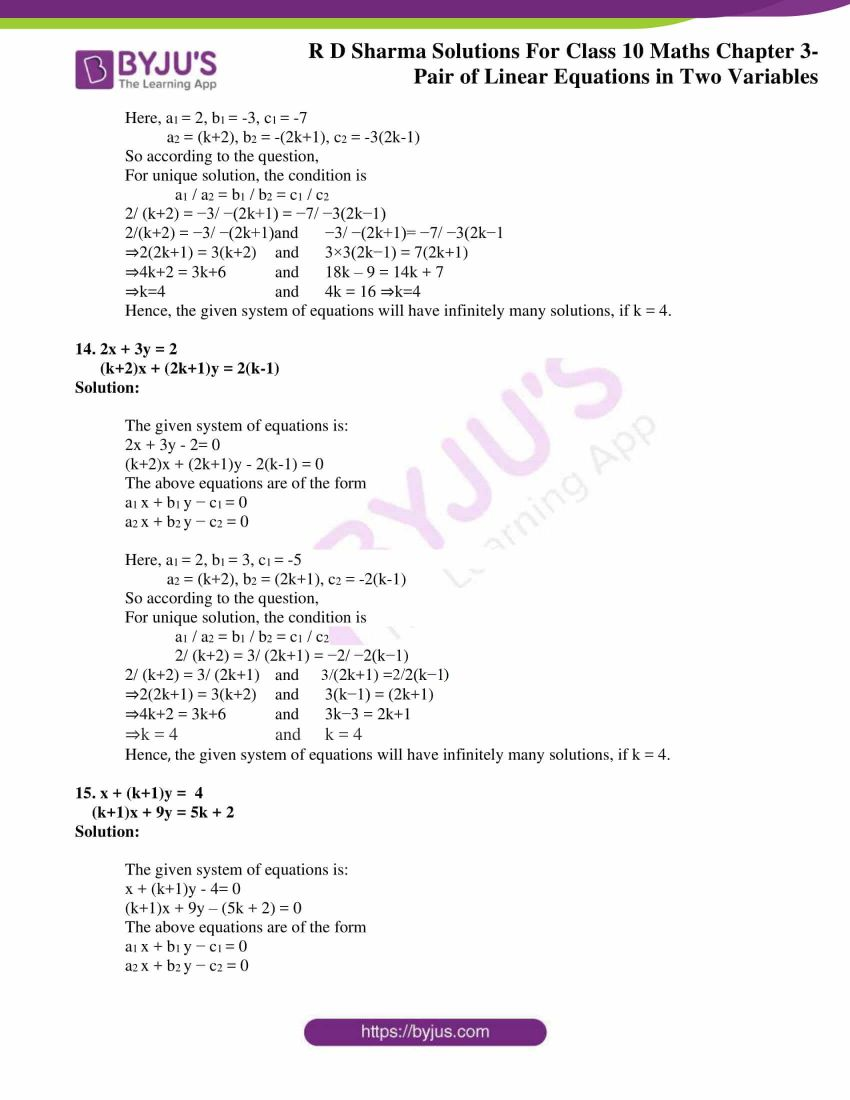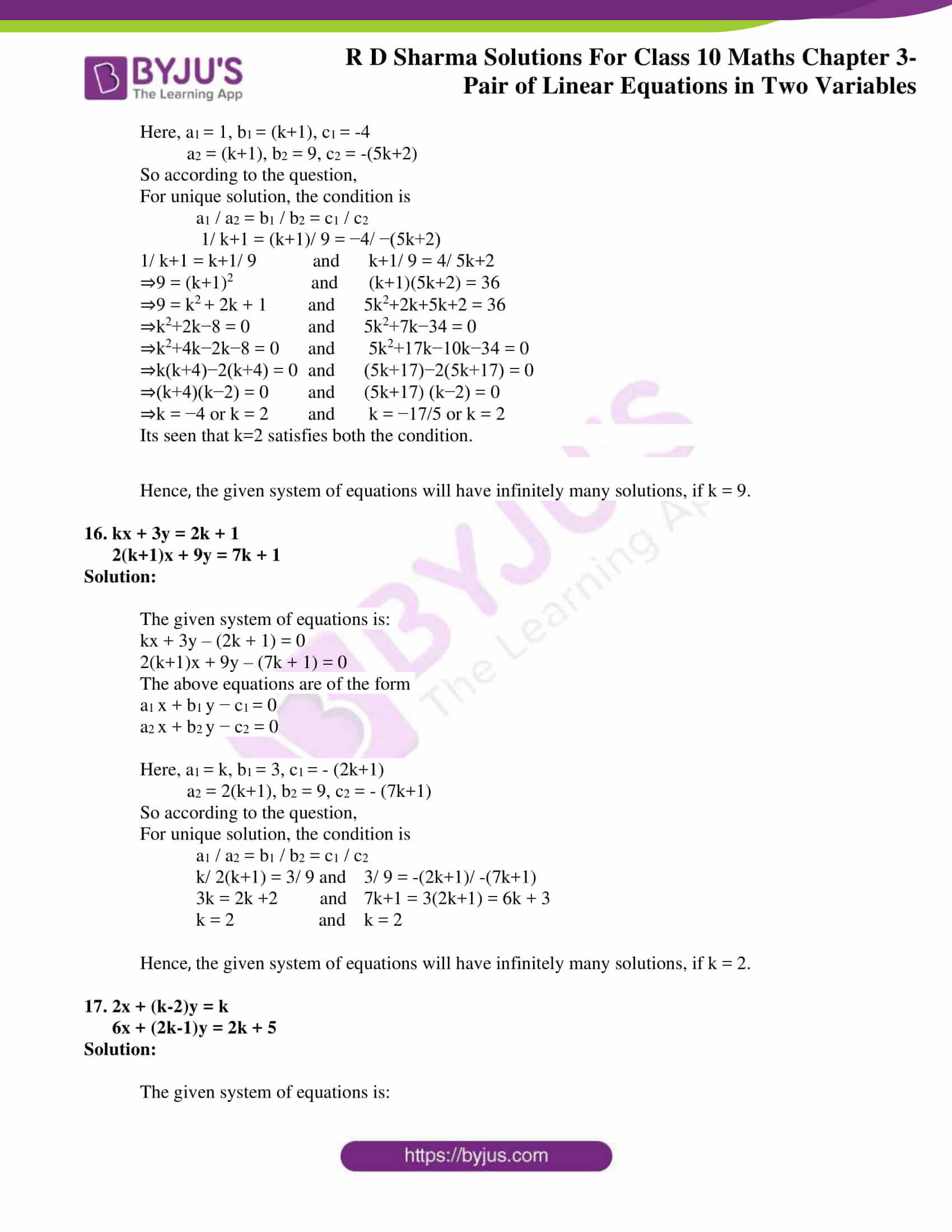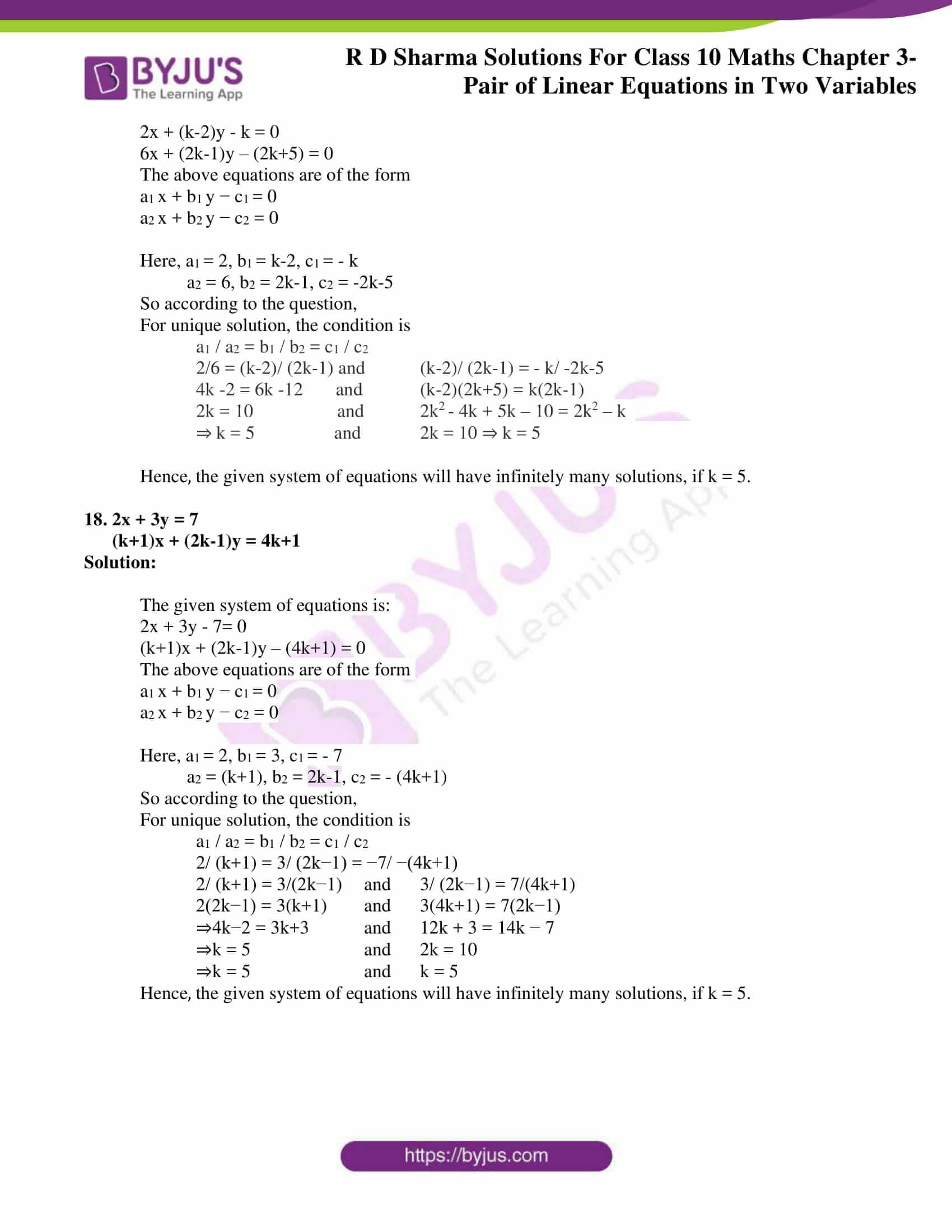### Access RD Sharma Solutions for Class 10 Maths Chapter 3 Pair of Linear Equations in Two Variables Exercise 3.5

In each of the following systems of equations, determine whether the system has a unique solution, no solution or infinite solutions. In case there is a unique solution, find it from 1 to 4:

1. x − 3y = 3

3x − 9y = 2

Solution:

The given system of equations is

x − 3y – 3 = 0

3x − 9y − 2 = 0

The above equations are of the form

a1 x + b1 y − c1 = 0

a2 x + b2 y − c2 = 0

Here, a1 = 1, b1 = −3, c1 = −3

a2 = 3, b2 = −9, c2 = −2

So, according to the question, we get

a1 / a2 = 1/3

b1 / b2 = −3/ −9 = 1/3 and,

c1 / c2 = −3/ −2 = 3/2

⇒ a1 / a2 = b1/ b2 ≠ c1 / c2

Hence, we can conclude that the given system of equations has no solution.

2. 2x + y = 5

4x + 2y = 10

Solution:

The given system of equations is

2x + y – 5 = 0

4x + 2y – 10 = 0

The above equations are of the form

a1 x + b1 y − c1 = 0

a2 x + b2 y − c2 = 0

Here, a1 = 2, b1 = 1, c1 = −5

a2 = 4, b2 = 2, c2 = −10

So, according to the question, we get

a1 / a2 = 2/4 = 1/2

b1 / b2 = 1/ 2 and,

c1 / c2 = −5/ −10 = 1/2

⇒ a1 / a2 = b1/ b2 = c1 / c2

Hence, we can conclude that the given system of equations has infinitely many solutions.

3. 3x – 5y = 20

6x – 10y = 40

Solution:

The given system of equations is

3x – 5y – 20 = 0

6x – 10y – 40 = 0

The above equations are of the form

a1 x + b1 y − c1 = 0

a2 x + b2 y − c2 = 0

Here, a1 = 3, b1 = -5, c1 = −20

a2 = 6, b2 = -10, c2 = −40

So, according to the question, we get

a1 / a2 = 3/6 = 1/2

b1 / b2 = -5/ -10 = 1/2 and,

c1 / c2 = -20/ −40 = 1/2

⇒ a1 / a2 = b1/ b2 = c1 / c2

Hence, we can conclude that the given system of equations has infinitely many solutions.

4. x – 2y = 8

5x – 10y = 10

Solution:

The given system of equations is

x – 2y – 8 = 0

5x – 10y – 10 = 0

The above equations are of the form

a1 x + b1 y − c1 = 0

a2 x + b2 y − c2 = 0

Here, a1 = 1, b1 = -2, c1 = −8

a2 = 5, b2 = -10, c2 = -10

So, according to the question, we get

a1 / a2 = 1/5

b1 / b2 = -2/ -10 = 1/5 and,

c1 / c2 = -8/ −10 = 4/5

⇒ a1 / a2 = b1/ b2 ≠ c1 / c2

Hence, we can conclude that the given system of equations has no solution.

Find the value of k for which the following system of equations has a unique solution: (5-8)

5. kx + 2y = 5

3x + y = 1

Solution:

The given system of equations is

kx + 2y – 5 = 0

3x + y – 1 = 0

The above equations are of the form

a1 x + b1 y − c1 = 0

a2 x + b2 y − c2 = 0

Here, a1 = k, b1 = 2, c1 = −5

a2 = 3, b2 = 1, c2 = -1

So, according to the question,

For a unique solution, the condition is

a1 / a2 ≠ b1 / b2

k/3 ≠ 2/1

⇒ k ≠ 6

Hence, the given system of equations will have a unique solution for all real values of k other than 6.

6. 4x + ky + 8 = 0

2x + 2y + 2 = 0

Solution:

The given system of equations is

4x + ky + 8 = 0

2x + 2y + 2 = 0

The above equations are of the form

a1 x + b1 y − c1 = 0

a2 x + b2 y − c2 = 0

Here, a1 = 4, b1 = k, c1 = 8

a2 = 2, b2 = 2, c2 = 2

So, according to the question,

For a unique solution, the condition is

a1 / a2 ≠ b1 / b2

4/2 ≠ k/2

⇒ k ≠ 4

Hence, the given system of equations will have a unique solution for all real values of k other than 4.

7. 4x – 5y = k

2x – 3y = 12

Solution

The given system of equations is

4x – 5y – k = 0

2x – 3y – 12 = 0

The above equations are of the form

a1 x + b1 y − c1 = 0

a2 x + b2 y − c2 = 0

Here, a1 = 4, b1 = 5, c1 = -k

a2 = 2, b2 = 3, c2 = 12

So, according to the question,

For a unique solution, the condition is

a1 / a2 ≠ b1 / b2

4/2 ≠ 5/3

⇒k can have any real values.

Hence, the given system of equations will have a unique solution for all real values of k.

8. x + 2y = 3

5x + ky + 7 = 0

Solution:

The given system of equations is

x + 2y – 3 = 0

5x + ky + 7 = 0

The above equations are of the form

a1 x + b1 y − c1 = 0

a2 x + b2 y − c2 = 0

Here, a1 = 1, b1 = 2, c1 = -3

a2 = 5, b2 = k, c2 = 7

So, according to the question,

For a unique solution, the condition is

a1 / a2 ≠ b1 / b2

1/5 ≠ 2/k

⇒ k ≠ 10

Hence, the given system of equations will have a unique solution for all real values of k other than 10.

Find the value of k for which each of the following systems of equations has infinitely many solutions. (9-19)

9. 2x + 3y – 5 = 0

6x + ky – 15 = 0

Solution:

The given system of equations is

2x + 3y – 5 = 0

6x + ky – 15 = 0

The above equations are of the form

a1 x + b1 y − c1 = 0

a2 x + b2 y − c2 = 0

Here, a1 = 2, b1 = 3, c1 = -5

a2 = 6, b2 = k, c2 = -15

So, according to the question,

For a unique solution, the condition is

a1 / a2 = b1 / b2 = c1 / c2

2/6 = 3/k

⇒ k = 9

Hence, the given system of equations will have infinitely many solutions, if k = 9.

10. 4x + 5y = 3

kx + 15y = 9

Solution:

The given system of equations is

4x + 5y – 3= 0

kx + 15y – 9 = 0

The above equations are of the form

a1 x + b1 y − c1 = 0

a2 x + b2 y − c2 = 0

Here, a1 = 4, b1 = 5, c1 = -3

a2 = k, b2 = 15, c2 = -9

So, according to the question,

For a unique solution, the condition is

a1 / a2 = b1 / b2 = c1 / c2

4/ k = 5/ 15 = −3/ −9

4/ k = 1/ 3

⇒k = 12

Hence, the given system of equations will have infinitely many solutions, if k = 12.

11. kx – 2y + 6 = 0

4x – 3y + 9 = 0

Solution:

The given system of equations is

kx – 2y + 6 = 0

4x – 3y + 9 = 0

The above equations are of the form

a1 x + b1 y − c1 = 0

a2 x + b2 y − c2 = 0

Here, a1 = k, b1 = -2, c1 = 6

a2 = 4, b2 = -3, c2 = 9

So, according to the question,

For a unique solution, the condition is

a1 / a2 = b1 / b2 = c1 / c2

k/ 4 = −2/ −3 = 2/ 3

⇒k = 8/ 3

Hence, the given system of equations will have infinitely many solutions, if k = 8/3.

12. 8x + 5y = 9

kx + 10y = 18

Solution:

The given system of equations is

8x + 5y – 9 = 0

kx + 10y – 18 = 0

The above equations are of the form

a1 x + b1 y − c1 = 0

a2 x + b2 y − c2 = 0

Here, a1 = 8, b1 = 5, c1 = -9

a2 = k, b2 = 10, c2 = -18

So, according to the question,

For a unique solution, the condition is

a1 / a2 = b1 / b2 = c1 / c2

8/k = 5/10 = −9/ −18 = 1/2

⇒k=16

Hence, the given system of equations will have infinitely many solutions, if k = 16.

13. 2x – 3y = 7

(k+2)x – (2k+1)y = 3(2k-1)

Solution:

The given system of equations is

2x – 3y – 7 = 0

(k+2)x – (2k+1)y – 3(2k-1) = 0

The above equations are of the form

a1 x + b1 y − c1 = 0

a2 x + b2 y − c2 = 0

Here, a1 = 2, b1 = -3, c1 = -7

a2 = (k+2), b2 = -(2k+1), c2 = -3(2k-1)

So, according to the question,

For a unique solution, the condition is

a1 / a2 = b1 / b2 = c1 / c2

2/ (k+2) = −3/ −(2k+1) = −7/ −3(2k−1)

2/(k+2) = −3/ −(2k+1)and −3/ −(2k+1)= −7/ −3(2k−1

⇒2(2k+1) = 3(k+2) and 3×3(2k−1) = 7(2k+1)

⇒4k+2 = 3k+6 and 18k – 9 = 14k + 7

⇒k=4 and 4k = 16 ⇒k=4

Hence, the given system of equations will have infinitely many solutions, if k = 4.

14. 2x + 3y = 2

(k+2)x + (2k+1)y = 2(k-1)

Solution:

The given system of equations is

2x + 3y – 2= 0

(k+2)x + (2k+1)y – 2(k-1) = 0

The above equations are of the form

a1 x + b1 y − c1 = 0

a2 x + b2 y − c2 = 0

Here, a1 = 2, b1 = 3, c1 = -5

a2 = (k+2), b2 = (2k+1), c2 = -2(k-1)

So, according to the question,

For a unique solution, the condition is

a1 / a2 = b1 / b2 = c1 / c2

2/ (k+2) = 3/ (2k+1) = −2/ −2(k−1)

2/ (k+2) = 3/ (2k+1) and 3/(2k+1) = 2/2(k−1)

⇒2(2k+1) = 3(k+2) and 3(k−1) = (2k+1)

⇒4k+2 = 3k+6 and 3k−3 = 2k+1

⇒k = 4 and k = 4

Hence, the given system of equations will have infinitely many solutions, if k = 4.

15. x + (k+1)y = 4

(k+1)x + 9y = 5k + 2

Solution:

The given system of equations is:

x + (k+1)y – 4= 0

(k+1)x + 9y – (5k + 2) = 0

The above equations are of the form

a1 x + b1 y − c1 = 0

a2 x + b2 y − c2 = 0

Here, a1 = 1, b1 = (k+1), c1 = -4

a2 = (k+1), b2 = 9, c2 = -(5k+2)

So, according to the question,

For a unique solution, the condition is

a1 / a2 = b1 / b2 = c1 / c2

1/ k+1 = (k+1)/ 9 = −4/ −(5k+2)

1/ k+1 = k+1/ 9 and k+1/ 9 = 4/ 5k+2

⇒9 = (k+1)2 and (k+1)(5k+2) = 36

⇒9 = k2 + 2k + 1 and 5k2+2k+5k+2 = 36

⇒k2+2k−8 = 0 and 5k2+7k−34 = 0

⇒k2+4k−2k−8 = 0 and 5k2+17k−10k−34 = 0

⇒k(k+4)−2(k+4) = 0 and (5k+17)−2(5k+17) = 0

⇒(k+4)(k−2) = 0 and (5k+17) (k−2) = 0

⇒k = −4 or k = 2 and k = −17/5 or k = 2

It’s seen that k=2 satisfies both conditions.

Hence, the given system of equations will have infinitely many solutions, if k = 9.

16. kx + 3y = 2k + 1

2(k+1)x + 9y = 7k + 1

Solution:

The given system of equations is

kx + 3y – (2k + 1) = 0

2(k+1)x + 9y – (7k + 1) = 0

The above equations are of the form

a1 x + b1 y − c1 = 0

a2 x + b2 y − c2 = 0

Here, a1 = k, b1 = 3, c1 = – (2k+1)

a2 = 2(k+1), b2 = 9, c2 = – (7k+1)

So, according to the question,

For a unique solution, the condition is

a1 / a2 = b1 / b2 = c1 / c2

k/ 2(k+1) = 3/ 9 and 3/ 9 = -(2k+1)/ -(7k+1)

3k = 2k +2 and 7k+1 = 3(2k+1) = 6k + 3

k = 2 and k = 2

Hence, the given system of equations will have infinitely many solutions, if k = 2.

17. 2x + (k-2)y = k

6x + (2k-1)y = 2k + 5

Solution:

The given system of equations is

2x + (k-2)y – k = 0

6x + (2k-1)y – (2k+5) = 0

The above equations are of the form

a1 x + b1 y − c1 = 0

a2 x + b2 y − c2 = 0

Here, a1 = 2, b1 = k-2, c1 = – k

a2 = 6, b2 = 2k-1, c2 = -2k-5

So, according to the question,

For a unique solution, the condition is

a1 / a2 = b1 / b2 = c1 / c2

2/6 = (k-2)/ (2k-1) and (k-2)/ (2k-1) = – k/ -2k-5

4k -2 = 6k -12 and (k-2)(2k+5) = k(2k-1)

2k = 10 and 2k2 – 4k + 5k – 10 = 2k2 – k

⇒ k = 5 and 2k = 10 ⇒ k = 5

Hence, the given system of equations will have infinitely many solutions, if k = 5.

18. 2x + 3y = 7

(k+1)x + (2k-1)y = 4k+1

Solution:

The given system of equations is

2x + 3y – 7= 0

(k+1)x + (2k-1)y – (4k+1) = 0

The above equations are of the form

a1 x + b1 y − c1 = 0

a2 x + b2 y − c2 = 0

Here, a1 = 2, b1 = 3, c1 = – 7

a2 = (k+1), b2 = 2k-1, c2 = – (4k+1)

So, according to the question,

For a unique solution, the condition is

a1 / a2 = b1 / b2 = c1 / c2

2/ (k+1) = 3/ (2k−1) = −7/ −(4k+1)

2/ (k+1) = 3/(2k−1) and 3/ (2k−1) = 7/(4k+1)

2(2k−1) = 3(k+1) and 3(4k+1) = 7(2k−1)

⇒4k−2 = 3k+3 and 12k + 3 = 14k − 7

⇒k = 5 and 2k = 10

⇒k = 5 and k = 5

Hence, the given system of equations will have infinitely many solutions, if k = 5.